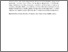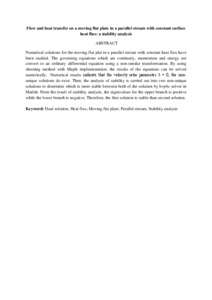# Flow and heat transfer on a moving flat plate in a parallel stream with constant surface heat flux: a stability analysis

## Citation

Ismail, Nurul Syuhada and Md Arifin, Norihan and Bachok @ Lati, Norfifah and Mahiddin, Norhasimah (2016) Flow and heat transfer on a moving flat plate in a parallel stream with constant surface heat flux: a stability analysis. Indian Journal of Science and Technology, 9 (31). pp. 1-5. ISSN 0974-6846; ESSN: 0974-5645

## Abstract

Numerical solutions for the moving flat plat in a parallel stream with constant heat flux have been studied. The governing equations which are continuity, momentum and energy are convert to an ordinary differential equation using a non-similar transformation. By using shooting method with Maple implementation, the results of the equations can be solved numerically. Numerical results indicate that the velocity ratio parameter λ < 0, the non-unique solutions do exist. Then, the analysis of stability is carried out into two non-unique solutions to determine which is more stable between both of the solution by bvp4c solver in Matlab. From the result of stability analysis, the eigenvalues for the upper branch is positive while the lower branch is negative. Therefore, the first solution is stable than second solution.Preview
Text
Flow and heat transfer on a moving flat plate in a parallel stream with constant surface heat flux.pdfView Item